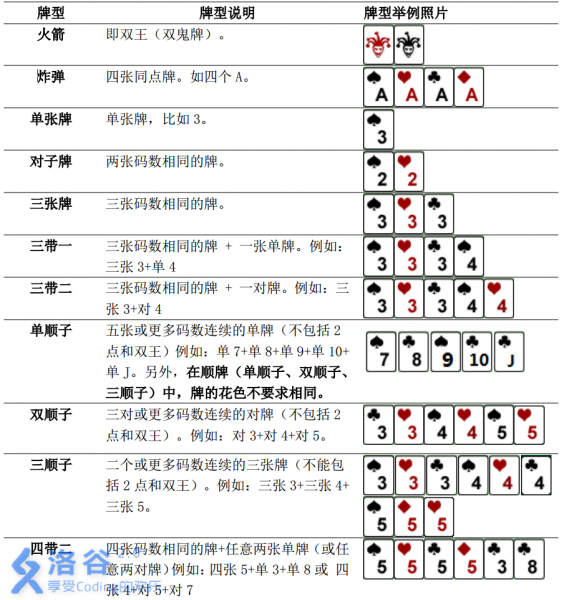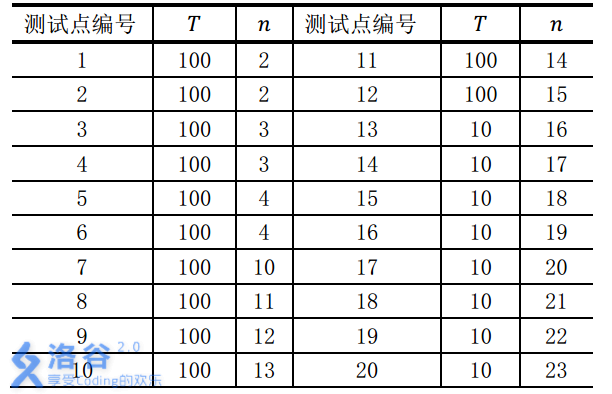P2668 斗地主

• 3.9K通过
• 16.3K提交
• 题目提供者 CCF_NOI
• 评测方式 云端评测
• 标签 搜索 深度优先搜索,DFS 贪心 NOIp提高组 2015
• 难度 提高+/省选-
• 时空限制 2000ms / 1024MB
• 提示：收藏到任务计划后，可在首页查看。

题目描述

牛牛最近迷上了一种叫斗地主的扑克游戏。斗地主是一种使用黑桃、红心、梅花、方片的$A$到$K$加上大小王的共$54$张牌来进行的扑克牌游戏。在斗地主中，牌的大小关 系根据牌的数码表示如下：$3<4<5<6<7<8<9<10<J<Q<K<A<2<\text{小王}<\text{大王}$，而花色并不对牌的大小产生影响。每一局游戏中，一副手牌由 $n$ 张牌组成。游戏者每次可以根据规定的牌型进行出牌，首先打光自己的手牌一方取得游戏的胜利。

现在，牛牛只想知道，对于自己的若干组手牌，分别最少需要多少次出牌可以将它们打光。请你帮他解决这个问题。

需要注意的是，本题中游戏者每次可以出手的牌型与一般的斗地主相似而略有不同。具体规则如下：本题数据随机，不支持hack，要hack或强力数据请点击这里

输入输出格式

输入格式：

第一行包含用空格隔开的2个正整数 $T,n$ ，表示手牌的组数以及每组手牌的张数。

接下来 $T$ 组数据，每组数据 $n$ 行，每行一个非负整数对 $a_i,b_i$ ，表示一张牌，其中 $a_i$ 表示牌的数码， $b_i$ 表示牌的花色，中间用空格隔开。特别的，我们用 $1$ 来表示数码 $A$， $11$ 表示数码$J$， $12$ 表示数码$Q$， $13$ 表示数码 $K$；黑桃、红心、梅花、方片分别用 $1-4$ 来表示；小王的表示方法为 $01$ ，大王的表示方法为 $02$ 。

输出格式：

共 $T$ 行，每行一个整数，表示打光第 $i$ 组手牌的最少次数。

输入输出样例

输入样例#1： 复制
1 8
7 4
8 4
9 1
10 4
11 1
5 1
1 4
1 1
输出样例#1： 复制
3

输入样例#2： 复制
1 17
12 3
4 3
2 3
5 4
10 2
3 3
12 2
0 1
1 3
10 1
6 2
12 1
11 3
5 2
12 4
2 2
7 2

输出样例#2： 复制
6


说明

样例1说明

共有$1$组手牌，包含8张牌：方片$7$，方片$8$，黑桃$9$，方片$10$，黑桃$J$，黑桃$5$，方片$A$以及黑桃$A$。可以通过打单顺子（方片$7$，方片$8$，黑桃$9$，方片$10$，黑桃$J$），单张牌（黑桃$5$）以及对子牌（黑桃$A$以及方片$A$）在$3$次内打光。

对于不同的测试点， 我们约定手牌组数$T$与张数$n$的规模如下：数据保证：所有的手牌都是随机生成的。

提示
标程仅供做题后或实在无思路时参考。
请自觉、自律地使用该功能并请对自己的学习负责。
如果发现恶意抄袭标程，将按照I类违反进行处理。